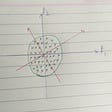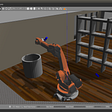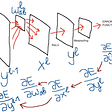# OneFlow’s Optimization of CUDA Elementwise Template Library: Practical, Efficient, and Extensible

Written by Zekang Zheng, Chi Yao, Ran Guo, Juncheng Liu; Translated by Xiaozhen Liu, Hengrui Zhang

Elementwise operation refers to applying a function transformation to every element of a tensor. In deep learning, many operators can be regraded as elementwise operators, such as common activation functions (like ReLU and GELU) and ScalarMultiply (multiplying each element of a tensor by a scalar).

For this elementwise operation, OneFlow abstracts a CUDA template.

In this article, we will introduce the design thoughts and optimization techniques of CUDA template.

OneFlow’s CUDA template helps developers get a CUDA Elementwise operator only by encapsulating the computational logic into a structure. Take ReLU as an example:

Such an Elementwise template is easy to use. It not only improves the developing efficiency but also ensures computing performance. We used Nsight Compute on the NVIDIA A100 40GB GPU to compare with the Cast operator of PyTorch. The testing example is to cast a tensor from `float32` to `half` type. The runtime and bandwidth show that for each data shape, OneFlow performs better than PyTorch by 80-90% and its bandwidth gets very close to its theoretical limit.

Next, we will introduce the design thoughts and optimization techniques of this template.

# Deciding the Optimal Block Size and Grid Size

For the number of blocks and threads, see How to Decide the Optimal grid_size and block_size in CUDA Kernel. But here the rules are slightly different. In CUDA official document Compute Capabilities, it was mentioned that:

• For mainstream architectures, the maximum number of 32-bit registers per thread block is 64 KB
• The maximum number of 32-bit registers per thread is 255

On the premise of using the maximum number of registers, each block can start up to `64 * 1024 / 255 = 256` threads (rounded to the multiple of 2). Therefore, here, we set a constant `constexpr int kBlockSize = 256;`.

For Grid Size, the rules are in the function `GetNumBlocks`:

• The minimum number of thread blocks is 1
• The maximum number of thread blocks is the minimum value between “the minimum total number of threads required to process all elements” and “the number of waves * the number of SM that can be scheduled by GPU at one time * the maximum number of blocks per SM”. Here, the number of waves is set to a fixed 32

When the amount of data is small, too many thread blocks will not be started. When the amount of data is large, we set the number of thread blocks to a sufficient integer multiple of waves to ensure the actual utilization of GPU is high enough.

# Using Vectorizing Operations

The computational logic of most Elementwise operators is simple and the pain point is on bandwidth utilization. In NVIDIA’s blog CUDA Pro Tip: Increase Performance with Vectorized Memory Access, it is mentioned that vectorizing operations can improve the read/write bandwidth utilization. A series of data types in the CUDA kernel are provided to support such as `float2` and `float4`, which take 2 or 4 float data as a whole. In some high-performance training and inference libraries like `LightSeq`, a large number of `float4` types are used:

In practice, the operators need to support different data types (such as int and half). If we use the built-in vectorizing operations of CUDA for data type, it is obvious that we need to write multiple versions of each operator, which increases developing burden. Therefore, we have invented a `Pack` data structure to flexibly support vectorization of different data types.

We first define a `PackType` to represent vectorized data. The data size it represents (after vectorization) is `sizeof(T) * pack_size`.

Then we introduce a `Pack` of `union` type, which internally defines `PackType<T, pack_size> storage;` to occupy space:

`T elem[pack_size];` shares the space with `storage`. This facilitates later Elementwise operations: in subsequent calculations, we apply `functor` to each element in the `elem` array to obtain the output result.

CUDA supports a maximum 128 bit pack size, while among floating-point data types, the minimum type (half) size is 16 bit, which can pack `128/16 = 8` half data together at most. Therefore, we set two constants: `kMaxPackBytes` represents the maximum number of bytes of the pack and `kMaxPackSize` represents the maximum number of pack data.

# Calling Chain

When tracking the implementation in `oneflow/core/cuda/elementwise.cuh`, you will find that this template provides interfaces called `Unary`, `Binary`, `Ternary`, for elementwise operators of one element, two elements, and three elements.

At the beginning of the article, the `ReLU` operator uses the interface of `Unary`. After further analysis, we can find that `ApplyGeneric` will eventually be called after layers of calls. The basic calling relationship is as follows:

The main work done in CUDA Kernel `ApplyGeneric` is:

• Create a `functor` based on the parameters
• Enter the loop, call `ApplyPack` function for the packed data, and process a batch of packed data every time `ApplyPack` is called
• When the number of elements cannot be divisible by `pack_size`, the remaining elements need to be processed by the thread

The code is as follows:

The `ApplyPack` function is defined as follows. It loops the elements in a `pack`, calls `functor` on each element in the `elem` array, gets the output result and returns:

The entire `Elementwise` operator calling process is as follows:

# Optimizing half2 Data Type

For the `half` data type, if it is directly operated on, its operator bandwidth is equivalent to that of `float32` type.

CUDA officially launched a series of special instructions for `half2`. For example, `hadd2` can add two `half2` data, thereby improving throughput.

In this situation, OneFlow specializes `ApplyPack` function to call special instructions related to `half2` by calling functor's `apply2` function. The interface is as follows:

Taking the `Cast` operator as an example, we call the `__float22half2_rn` instruction inside the `CastFunctor` to convert a `float2` data into a `half2` data.

# Expanding Multiple Operations

As mentioned above, the existing OneFlow templates further divides `Elementwise` operators into unary, binary, and ternary operations. By using the factory method pattern, The operators can call `ApplyGeneric` uniformly at last.

This design method is easy to expand, since developers only need to write the corresponding factory method when supporting more input operations.

This article mainly introduces OneFlow’s design and optimization methods of a high-performance CUDA `Elementwise` template. At last, let’s summarize the advantages of this template:

1. High performance. Operators using this `Elementwise` template can fill the bandwidth of the machine, and the speed is fast enough.
2. High development efficiency. Developers don’t need to pay too much attention to CUDA logic and related optimization methods, but only need to write calculation logic.
3. Strong scalability. Currently, this template supports unary, binary, and ternary operations. Developers only need to write the corresponding factory method if there is a need to expand to support more inputs.

Related articles：

Also, welcome to join our Discord group to discuss and ask OneFlow related questions, and connect with OneFlow contributors and users all around the world.

--

--

--

## More from OneFlow

OneFlow is a performance-centered and open-source deep learning framework.

Love podcasts or audiobooks? Learn on the go with our new app.

## Deep learning based sentiment analysis of drug reviews## Dimensionality Reduction and Visualization - Part-4 - tSNE## Robotic Arm/ Pick & Place## Applications Of Machine Learning## Composing Deep-Learning Microservices for the Hybrid Internet of Things## Introduction to Computer vision with AWS and Python## CNN in Python, part 1. Defining the main parameters of the model## OneFlow

OneFlow is a performance-centered and open-source deep learning framework.

## The History of Credit-based Flow Control (Part 1)## How to train a Graph Convolutional Network on the Cora dataset with PyTorch Geometric## A little thinking on avoiding GPU memory outage during the model training (PyTorch)## The FLOPs Calculus of Language Model Training